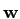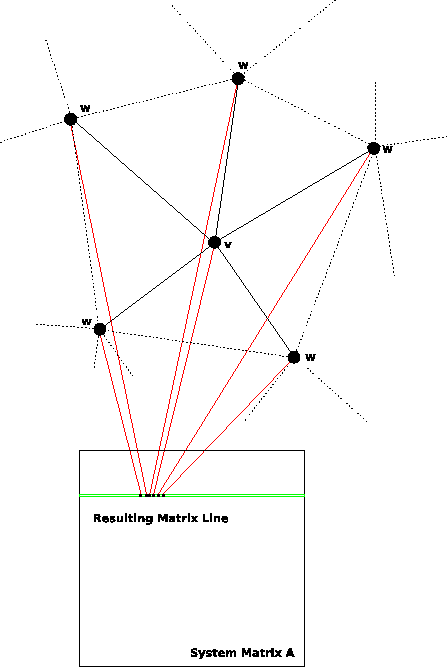## 4. 2. 1 Comparison to Line-Wise Assembly

In many cases element-wise assembly requires less numerical costs to assemble, because cell-related quantities can be calculated together and need not be re-calculated in each step. However, most of the cell-wise calculations can be stored into the cells as a pre-processing step.

Another feature which makes the element-wise assembly faster than line-wise assembly is that the number of operations which have to be performed for a cell is better to predetermine, because cells usually have the same topological shape throughout the simulation domain. If the number of operations is constant, the compiler can perform various optimizations, e.g. perform parallel execution or handle this as a loop of fixed iterations.

For the parallel treatment of big equation systems the parallel assembly can be easier performed via line-wise application, because matrix insertions are performed line-wise and therefore no concurrent access methods are required.

Compared to element-wise assembly, line-wise assembly imposes higher requirements on the underlying data structures, while the matrix assembly is straight forward. For the implementation typically two traversal functions have to be available, for instance a function that yields all vertices incident to a given cell and another function that yields all cells incident to a given vertex. The assembly of the system matrix is rather simple, because the system matrix is separated into disjoint regions, namely lines, where each line can be assembled separately, especially using parallel computing mechanisms.

In contrast, element-wise assembly only requires one traversal function which provides all elements incident subsets of a given element, for instance the set of all vertices which are incident to a given cell. Therefore requires the possibility of inserting element sub-matrices or local matrices into the system matrix. In this case it is possible that two local matrices overlap. When using parallel processes for the assembly it is possible that two or more processes require access to the same matrix element which imposes various difficulties of synchronization and ressource management.

These different models of assembly lead to completely different matrix interfaces: Whereas for line-wise assembly it is only required to access each non-zero matrix element at most once, the element-wise access requires different operations, even deleting single matrix rows . Other solver interfaces require to add single matrix elements into the system matrix, where each matrix element can be accessed for several times [84,36,85]. For the sake of completeness, it has to be said, that the frameworks investigated for element-wise assembly do not require the topological operations which are needed for line-wise assembly. Figure 4.4 shows the application of line-wise assembly for a finite volume method with an initial vertexand five neighboring vertices. The assembly method yields one matrix line which is directly inserted into the system matrix.

Linear solver environments such as Trilinos  and PetSC  support element-wise access to single matrix entries. A matrix entry can either be added a given value or is overwritten. Furthermore, it is possible to insert local sub-matrices for optimized finite element assembly.Michael 2008-01-16